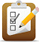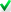US STOCKS
FUNDS
FX & CRYPTO
SH/SZ-HK
HK STOCKS

Schroder ISF-Hong Kong Equity (HKD) A Acc
 Chart Type |  Color - Full Screen BarMountainLine
Day  |  Week  |  MonthPeriod
Daily 1 Month ． 3 Months ．6 Months ． 1 Year ． 3 Years
Weekly 6 Months ． 1 Year ． 3 Years ． 8 Years
Monthly 3 Years ． 8 Years
Chart Setting
Type Bar ． Mountain ．Line
Background
Overlay Indicator
Indicator
None  WMASMAReset Draw Cancel
 WMARef. 1Ref. 2 Ref. 3 Ref. 4 Ref. 5 Reset Draw Cancel Description: The Weighted Moving Average (WMA) finds the average price of a security over a set number of periods. It gives more weight to the more recent prices, relative to older prices, in an attempt to reduce the lag associated with moving averages, in general. This is calculated by multiplying each of the previous interval's data by a weight based on the period length in the WMA. Each price is multiplied by a factor, with the current price having the highest factor and each price after that having a factor of the prior price less one. Common setting: Number of periods = 10, 20, 50, 100, 150
 SMARef. 1Ref. 2 Ref. 3 Ref. 4 Ref. 5 Reset Draw Cancel Description: Moving averages smooth the price data to form a trend following indicator. A simple moving average is formed by computing the average price of a security over a specific number of periods. Old data is dropped as new data comes available. This causes the average to move along the time scale. Common setting: Number of periods = 10, 20, 50, 100, 150
EMABollingerEMARef. 1Ref. 2 Ref. 3 Ref. 4 Ref. 5 Reset Draw Cancel Description: Exponential moving average is a type of moving average that is similar to a simple moving average, except that more weight is given to the latest data. The weighting for each older data point decreases exponentially, never reaching zero. It reduces the lag by applying more weight to recent prices. Common setting: Number of periods = 10, 20, 50, 100, 150
 BollingerRef. 1Ref. 2 Reset Draw Cancel Description: Bollinger Bands are volatility bands placed above and below a moving average. The Bollinger Band includes 3 lines: the upper band, lower band, and the centerline. The centerline is simply the moving average, and the upper and lower bands are, respectively, the center line plus/minus twice the standard deviation. Common setting: Centerline SMA = 20 Standard Deviation = 2
Technical Analysis (Display up to 4 subchart layers)
Clear All Layers
RSIMACDMTMRSIRef. 1Reset Draw Cancel Description: A technical momentum indicator that compares the magnitude of recent gains to recent losses in an attempt to determine overbought and oversold conditions of an asset. Common setting: Number of periods = 9, 14, 25
 MACDRef. 1Ref. 2 Ref. 3 Reset Draw Cancel Description: A trend-following momentum indicator that shows the relationship between two moving averages of prices. The MACD is calculated by subtracting the 26-day exponential moving average (EMA) from the 12-day EMA. A nine-day EMA of the MACD, called the "signal line", is then plotted on top of the MACD, functioning as a trigger for buy and sell signals. Common setting: Shorter EMA = 12 Longer EMA = 26 Signal line – EMA of MACD = 9
 MTMRef. 1Reset Draw Cancel Description: Momentum is an oscillator-type indicator used to detect overbought and oversold conditions and to perform as a gauge indicating the strength of the current trend. When momentum is above the zero-line and rising, prices are increasing at an increasing rate. If momentum is above the zero line but is declining, prices are still increasing but at a decreasing rate. On the other hand, when momentum is below the zero-line and falling, prices are decreasing at an increasing rate. If momentum is below the zero line but is rising, prices are still declining but at a decreasing rate. Common setting: Number of periods = 9
ROCROCRef. 1Reset Draw Cancel Description: It measures the percentage change in price over a given period of time. As a momentum oscillator, ROC signals include centerline crossovers, divergences and overbought-oversold readings. Common setting: Number of periods = 12
PeriodDaily 1 Month ． 3 Months ．6 Months ． 1 Year ． 3 YearsWeekly 6 Months ． 1 Year ． 3 Years ． 8 YearsMonthly 3 Years ． 8 Years
OverlayIndicator
 NoneWMASMAEMABollinger
Reset
Draw
Technical Analysis
Lower IndicatorLayer ILayer IILayer IIILayer IV